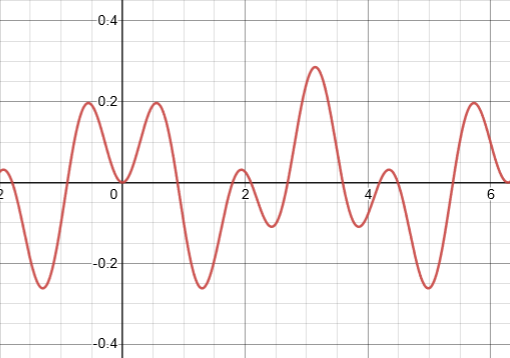## Tuesday, June 29, 2021

### Forced Oscillation 1: UNIZOR.COM - Physics4Teens - Waves - Mechanical Os...

Notes to a video lecture on http://www.unizor.com

Forced Oscillation 1

Oscillation is forced, when some periodic force is applied to an object that can potentially oscillate.

A subject of our analysis will be an object on a spring with no other forces acting on it except one external force that we assume is periodical.

As we know, in the absence of external force the differential equation describing the movement of an object on a spring follows from the Hooke's Law and the Newton's Second Law
m·x"(t) = −k·x(t)
or
m·x"(t) + k·x(t) = 0
with a general solution
x(t) = A·cos(ω0·t) + B·sin(ω0·t)
where
A and B are any constants;
ω0 = √k/m is an inherent natural angular frequency of oscillation of this particular object on this particular spring.

The equation above is homogeneous because, together with any its solution x(t), the function C·x(t) will be a solution as well.

Consider a model of forced oscillation of an object on a spring with a periodic external force acting on it with the following characteristics:
elasticity of a spring k,
mass of an object is m,
force applied to an object is F(t)=F0·cos(ω·t).

In this lecture we consider a case of angular frequency ω of the periodic external function F(t)=F0·cos(ω·t) to be different from the inherent natural angular frequency ω0=√k/m of oscillations without external forces:
ω ≠ ω0 = √k/m

If external force F(t), described above, is present, the differential equation that describes the oscillation of an object looks like
m·x"(t) = −k·x(t) + F(t)
or, assuming the external force is a periodic function F(t)=F0·cos(ω·t),
m·x"(t)+ k·x(t) = F0·cos(ω·t)
The function on the right of this equation makes this equation non-homogeneous.
Our task is to analyze the movement of an object on a spring in the presence of a periodic external function, as described by the above differential equations and initial conditions.

Notice that if some non-homogeneous linear differential equation has two partial solutions x1(t) and x2(t), their difference is a partial solution to a corresponding homogeneous linear differential equation.
Indeed, if
m·x1"(t)+ k·x1(t) = F0·cos(ω·t)
and
m·x2"(t)+ k·x2(t) = F0·cos(ω·t)
then for x3(t)=x1(t)−x2(t)
m·x3"(t)+ k·x3(t) = 0

From the above observation follows that, in order to find a general solution to a non-homogeneous linear differential equation, it is sufficient to find its one partial solution and add to it a general solution of a corresponding homogeneous equation.

In our case we already know the general solution to a corresponding homogeneous equation
m·x"(t)+ k·x(t) = 0
So, all we need is to find a single partial solution to
m·x"(t)+ k·x(t) = F0·cos(ω·t)
and add to it the general solution to the above homogeneous equation.

Considering our differential equation, that describes the forced oscillation with a periodic external function, contains function x(t), its second derivative and function cos(ω·t) on its right side, it's reasonable to look for a partial solution xp(t) in a form of xp(t)=C·cos(ω·t) and find such a coefficient C that the equation is satisfied.
Then
xp(t) = C·cos(ω·t)
x'p(t) = −C·ω·sin(ω·t)
x"p(t) = −C·ω²·cos(ω·t)
Substituting these into our differential equation, we get
−m·C·ω²·cos(ω·t) +
+ k·C·cos(ω·t) = F0·cos(ω·t)

Since this is supposed to be true for any time value t, the coefficients at cos(ω·t) must satisfy the equation:
−m·C·ω² + k·C = F0
from which follows
C = F0 /(k−m·ω²) =
= F0 /m·((k/m)−ω²) =
= F0 /
[m·(ω0²−ω²)]

We have found a partial solution to our non-homogeneous equation:
xp(t)=F0·cos(ω·t)/[m·(ω0²−ω²)]

Now we can express the general solution to a differential equation that describes the forced oscillation by a periodic external function as
x(t)=F0·cos(ω·t)/[m(ω0²−ω²)] +
+ A·cos(ω0·t) + B·sin(ω0·t)

where
A and B are any constants determined by initial conditions x(0) and x'(0);
ω0 = √k/m is an inherent natural angular frequency of oscillation of this particular object on this particular spring;
F0·cos(ω·t) is an external periodic force acting on an object.

It's beneficial to represent A·cos(ω0·t)+B·sin(ω0·t) through a single trigonometric function as follows.
Let
D = √A²+B²
Angle φ is defined by
cos(φ) = A/D
sin(φ) = B/D
(on a Cartesian coordinate plane this angle is the one from the X-axis to a vector with coordinates {A,B})
Then
A·cos(ω0·t)+B·sin(ω0·t) =
= D·
[cos(φ)·cos(ω0·t) +
+ sin(φ)·sin(ω0·t)
] =
= D·cos(ω0·t−φ)

Now the general solution to our differential equation that describes forced oscillation looks like
x(t)=F0·cos(ω·t)/[m(ω0²−ω²)] +
+ D·cos(ω0·t−φ)

where
F0 is an amplitude of a periodic external force;
ω - angular frequency of a periodic external force;
m - mass of an object on a spring;
k - elasticity of a spring;
ω0=√k/m is an inherent natural angular frequency of oscillation of this particular object on this particular spring;
t is time;
D and φ parameters are determined by initial conditions for x(0) and x'(0).

As you see from the formula for a general solution, it's essential that ω0≠ω, that is an angular frequency of the external force is not equal to an inherent natural angular frequency of the object and a spring in the absence of external force.
Their equality renders zero in the denominator of a formula, invalidating the whole approach.
What happens if these frequencies are equal is a subject of the next lecture.

The solution to a forced oscillation in a case of ω0≠ω represents a superposition of two sinusoidal functions with different frequencies.
Let's represent it graphically for some specific case of initial conditions and parameters.

Initial conditions:
x(0) = 0
x'(0) = 0
From these initial conditions follows
0 = F0/[m(ω0²−ω²)] + D·cos(φ)
0 = D·ω0·sin(φ)
The second equation results in φ=0, from which the first equation gives
D = −F0/[m(ω0²−ω²)]
Parameters:
m=1
k=4
ω0=√k/m=2
F0=3
ω=5
The parameters above define the value of D:
D=−3/(4−25)=1/7

With the above initial conditions and parameters the oscillation of the object is described by function
x(t)=−3·cos(5t)/21+cos(2t)/7 =
=
[cos(2t)−cos(5t)]/7

The graph of this function looks like this